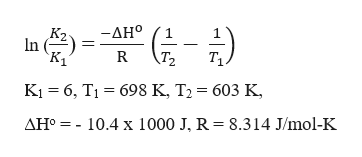# The equilibrium constant, Kp, for the following reaction is 6at 698 K.H2(g) + I2(g) 2HI(g)If ΔH° for this reaction is -10.4 kJ, what is the value of Kp at 603 K?Kp =

Question
11 views
1. The equilibrium constant, Kp, for the following reaction is 6at 698 K.

H2(g) + I2(g) 2HI(g)

If ΔH° for this reaction is -10.4 kJ, what is the value of Kp at 603 K?

Kp =
check_circle

Step 1

To calculate Kp at 603 K, the following expression can be used,help_outlineImage Transcriptionclose-AHo In Ti R T2 Ki 6, TI 698 K, T2 603 K AHo 10.4 x 1000 J, R = 8.314 J/mol-K fullscreen
Step 2

Substituting the values in the ...

### Want to see the full answer?

See Solution

#### Want to see this answer and more?

Solutions are written by subject experts who are available 24/7. Questions are typically answered within 1 hour.*

See Solution
*Response times may vary by subject and question.
Tagged in

### Chemical Thermodynamics